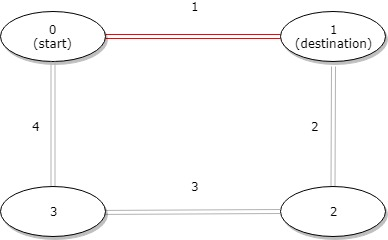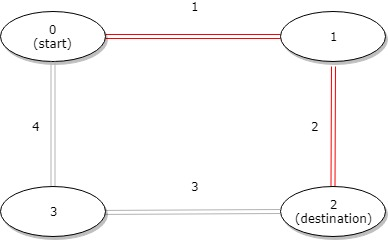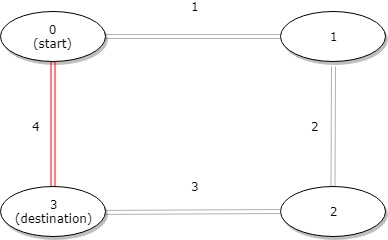## Algorithm

Problem Name: 1184. Distance Between Bus Stops

A bus has `n` stops numbered from `0` to `n - 1` that form a circle. We know the distance between all pairs of neighboring stops where `distance[i]` is the distance between the stops number `i` and `(i + 1) % n`.

The bus goes along both directions i.e. clockwise and counterclockwise.

Return the shortest distance between the given `start` and `destination` stops.

Example 1:```Input: distance = [1,2,3,4], start = 0, destination = 1
Output: 1
Explanation: Distance between 0 and 1 is 1 or 9, minimum is 1.```

Example 2:```Input: distance = [1,2,3,4], start = 0, destination = 2
Output: 3
Explanation: Distance between 0 and 2 is 3 or 7, minimum is 3.
```

Example 3:```Input: distance = [1,2,3,4], start = 0, destination = 3
Output: 4
Explanation: Distance between 0 and 3 is 6 or 4, minimum is 4.
```

Constraints:

• `1 <= n <= 10^4`
• `distance.length == n`
• `0 <= start, destination < n`
• `0 <= distance[i] <= 10^4`

## Code Examples

### #1 Code Example with Java Programming

```Code - Java Programming```

``````
class Solution {
public int distanceBetweenBusStops(int[] distance, int start, int destination) {
if (start == destination) {
return 0;
}
int n = distance.length;
int actualStart = Math.min(start, destination);
int actualDestination = Math.max(start, destination);
return Math.min(
getStraightDistance(distance, actualStart, actualDestination, n),
getReverseDistance(distance, actualDestination, actualStart, n)
);
}

private int getStraightDistance(int[] distance, int start, int end, int n) {
int dist = 0;
while (start != end) {
dist += distance[start++];
}
return dist;
}

private int getReverseDistance(int[] distance, int end, int start, int n) {
int dist = 0;
while (end != start) {
dist += distance[end++];
end = end % n;
}
return dist;
}
}
``````
Copy The Code &

Input

cmd
distance = [1,2,3,4], start = 0, destination = 1

Output

cmd
1

### #2 Code Example with Javascript Programming

```Code - Javascript Programming```

``````
const distanceBetweenBusStops = function(distance, start, destination) {
if (start > destination) {
let temp = start
start = destination
destination = temp
}
let res = 0,
total = 0
for (let i = 0; i < distance.length; i++) {
if (i >= start && i < destination) {
res += distance[i]
}
total += distance[i]
}
return Math.min(res, total - res)
}
``````
Copy The Code &

Input

cmd
distance = [1,2,3,4], start = 0, destination = 1

Output

cmd
1

### #3 Code Example with Python Programming

```Code - Python Programming```

``````
class Solution:
def distanceBetweenBusStops(self, d: List[int], i: int, j: int) -> int:
return min(sum(d[min(i, j):max(i, j)]), sum(d[:min(i, j)] + d[max(i, j):]))
``````
Copy The Code &

Input

cmd
distance = [1,2,3,4], start = 0, destination = 2

Output

cmd
3

### #4 Code Example with C# Programming

```Code - C# Programming```

``````
using System;

namespace LeetCode
{
public class _1184_DistanceBetweenBusStops
{
public int DistanceBetweenBusStops(int[] distance, int start, int destination)
{
if (start > destination)
{
var temp = start;
start = destination;
destination = temp;
}

int dist1 = 0, dist2 = 0;
for (int i = 0; i < distance.Length; i++)
{
if (i >= start && i < destination) dist2 += distance[i];
else dist1 += distance[i];
}

return Math.Min(dist1, dist2);
}
}
}
``````
Copy The Code &

Input

cmd
distance = [1,2,3,4], start = 0, destination = 2

Output

cmd
3